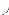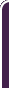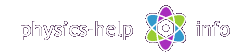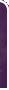homephysics online guidephysics help my info terms of use contactsWhat is Physics? Mechanics Electricity and Magnetism Electric Field Gauss' Law about below subjects Electric Potential Capacity Direct Current Magnetic Field Magnetic Field Laws Magnetic Interactions Electromagnetic Induction Maxwell's Equations Oscillations and Waves Simple Harmonic Motion Damped Harmonic Motion Driven Harmonic Motion Electric Oscillation Alternating Current Wave Motion Elastic Waves Electromagnetic Waves Optics Light Waves Geometrical Optics Interference Polarization Diffraction Fraunhofer Diffraction Dispersion, Absorption, Diffusion Doppler Effect Thermodynamics Ideal Gas Molecular Statistics Transport Phenomena First Law of Thermodynamics Second and Third Laws of Thermodynamics Imperfect Gas Liquids Solids Quantum Physics Thermal Radiation Quantum Properties of Light Wave Properties of Particles Planetary Model of Atom X-Rays Particle in Potential Well Pauli Exclusion Principle Nuclear Physics Solid State Physics Appendices# Gauss' Law   full version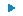SI units & Physics constants

The Gauss' law is a method, widely used to calculate the electric fields form symmetrically charged objects

## Electric fluxHere (all units see here):

A is area of small virtual plane inside the electric fieldis electric fieldis unit vector normal to the planeis angle between vectorsandThe electric flux thorough the area A is defined by scalar productThe net electric flux through any surface is defined by the sumwhere:

rea of small element i, for i = 1, 2, 3,..., n

n is total number of element over the surfaceis ais the electric field at the center of the element iis the angle betweenand the normal to the plane of the element i

Here the smaller valuethe more n, and so the more accurate value of the electric flux is defined

The exact value of net electric flux over a surface with area A is calculated by surface integralGauss surface for a given charges is any imaginary closed surface with area A, totally surrounding the chargesHereis electric field produced by the charges

The net enclosed charge inside the Gauss surface is defined aswhere n is total number of charges within the Gauss surface

## The Gauss' Law

The Gauss law states that electric net flux through any Gauss surface is directly proportional to the net charge enclosed by the surfacewhereis permittivity of vacuum

Electric field from uniformly charged thin spherical shell:outside the shellwith magnitudeinside the shellwhere:

Q is total charge of the shell

R is radius of the shellis position vector of point P where the electric field is defined

Electric field from uniformly charged solid sphere:

outside the spherewith magnitudeinside the spherewith magnitudeElectric field from uniformly charged thin line:with magnitudewhere:is linear charge density of the line with length L charged by charge Qis radius-vector drawn perpendicular to axis of the line from the axis to the point where the electric field is defined

Electric field from uniformly charged thin cylindrical shell:

outside the shellwith magnitudeinside the shellElectric field from uniformly charged solid cylinder:

outside the cylinderwith magnitudeinside the cylinderwith magnitudewhere:is linear charge density of the cylinder with length L charged by charge Q

R is radius of cylinderis radius-vector normal to axis of the line drawn from the axis to the point where the electric field is defined

Electric field near uniformly charged planewhereis surface charge density of the plane with area A charged by charge Q

## Conductors in electrostatic field

Electrostatic field is an electric field produced by fixed charges

The conductor is a substance with large number of free electrons which are not bounded to atoms and can freely move through entire substance under the influence of negligible small electric forces

The net electrostatic field inside the conductor is zeroHereis electrostatic field outside the conductor

A charge Q placed on a conductor is localised only over the surface of the conductor inside its thin surface layer AThe electrostatic field on the surface of a conductor is always directed perpendicular to the surface, so that its tangential component is zeroThe surface charge density of the conductor is defined by Gauss' Lawwhere E is electrostatic field on the surface

Electrostatic polarization of conductor

When an uncharged conductor is inserted into an external electrostatic field,, the electric field lines near the surface of the conductor are distorted, so that they are directed normal to the surface everywhere on the surface. As a result, the electriostatic field induces the opposite charges on the faces of the conductor as shown belowhome  |  physics online guide  |  physics help  |  my info  |  terms of use  |  contacts Dr. Nikitin © 2010